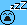# Plotting experimental results on resonant frequencies

ghdalswn Source htt
Homework Statement:
Using the value of μ, plot a graph based on the data from a table and vice versa.
Relevant Equations:
f=(n/2L) (√(T/μ)) (equation 1)

μ =T(n^2)/(4L^2)(f^2)

f frequency (Hz)
n Harmonic mode number (1,2,3,4,5)
L Length of string (1m)
T Tension of string (N)
μ linear mass density (mass per unit length)(kg/m)
These are the questions that I can't figure out how to do.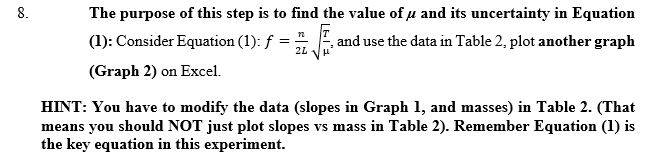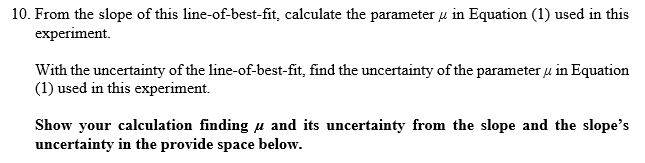I have been assigned a lab report based on resonating frequencies on a string and am having trouble completing some of the questions in the report. This is what i currently have from the previous steps required.

A bit of info regarding the experiment that was performed:
- A piece of string was attached to a vibration machine that will oscillate the string. The vibration machine is also connected to a signal generator that controls what frequency is chosen.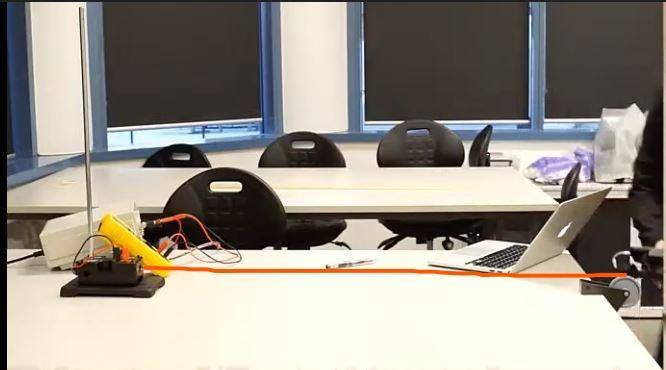- At the end of the string a mass is attached. (Starts from 0.1kg and increases to 0.5kg in 0.1kg increments). The length of the string from the vibration machine to the point of contact on the opposite side is 1m. (This is L in the experiment.)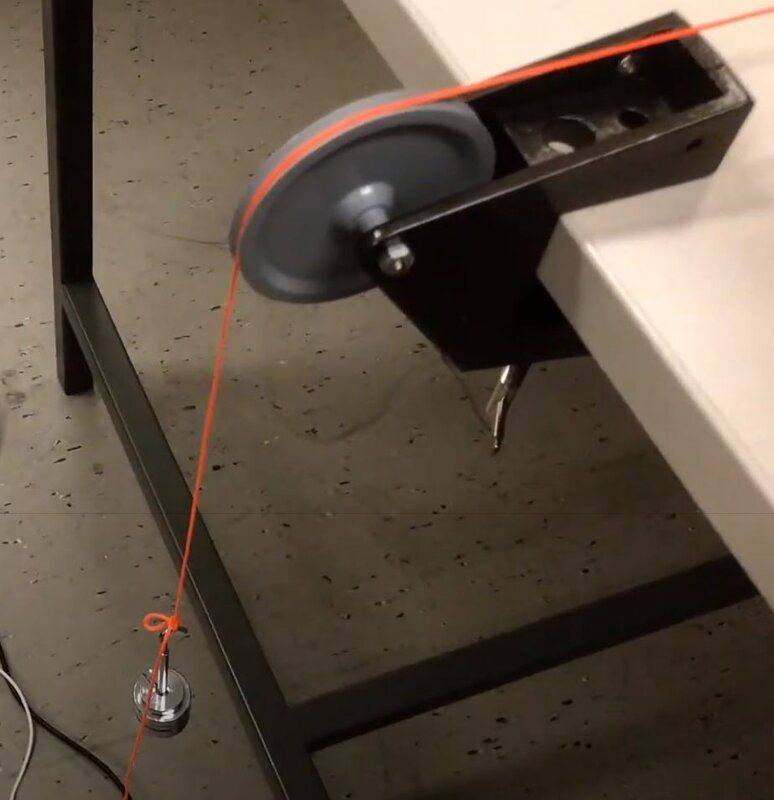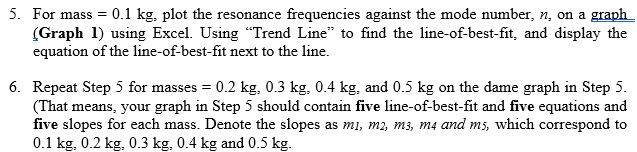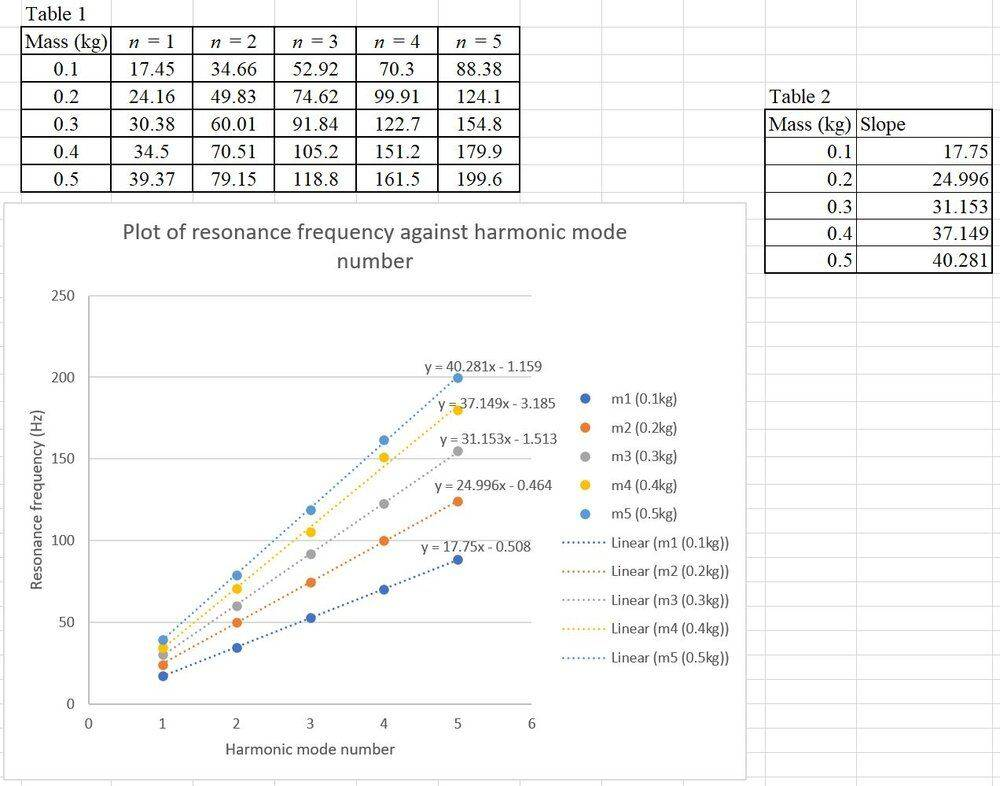I don't know what to do next. I tried finding the values of μ by re-arranging equation 1. I made μ the subject.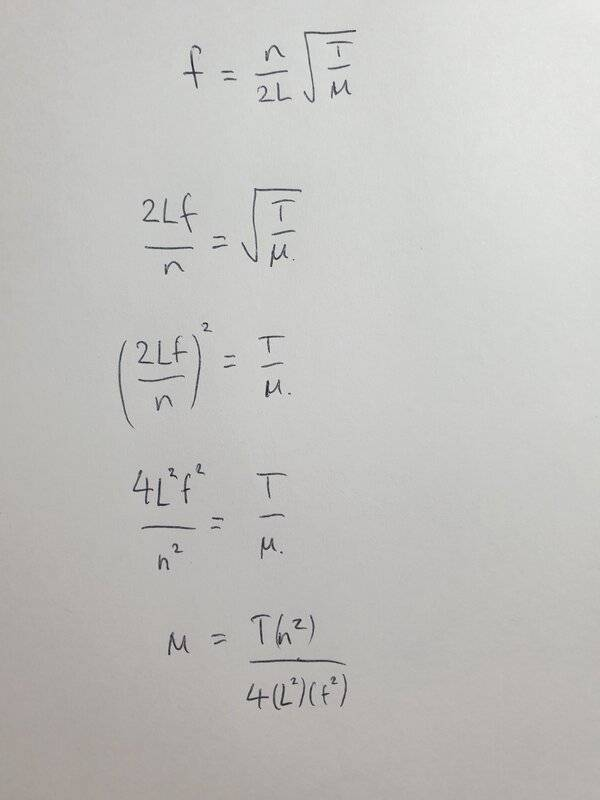So far i have (8.0 ± 0.5)10^-4 kg/m and (8.0 ± 0.9)10^-4 kg/m for m1 and m2. (m1 and m2 are the mass 0.1kg and 0.2kg respectively). I got these values by placing in the numbers, from table 1, into the formula i derived. I did this for all rows for mass 0.1kg(m1) and mass 0.2 kg(m2) to eventually find the best estimate (i added all the derived values and divided by 5) and the uncertainty (highest value - minus lowest value then divided by 5). However, i don't think this is the right direction as I didn't get how to link these values in regards to table 2.

I would be very thankful for any assistance.

#### Attachments

Last edited:

Homework Helper
Gold Member
2022 Award
It is not at all clear, but I think you are to rearrange the equation into the form ##y=\mu x##, where y is a function of some of T, L, f, n and x is a function of the rest. Then plot y against x.

Last edited:
Homework Helper
Hello ghd,!
by re-arranging equation 1
tell us how

I gather a plot of slope against mass is exactly what the hint say NOT to do. So why do you do it anyway ?

So far i have (8.0 ± 0.5)10^-4 kg/m and (8.0 ± 0.9)10^-4 kg/m for m1 and m2
Did you find them in a flower bed? The values themselves are unimprtant o us. Tell us how you obtained these values !

m1 and m2 are new players in your story -- they were not mentioned earlier. If I have to guess, they are ##\mu_1## and ##\mu_2## for the two wires for which the experiment was done. So I expect to see two plots 1 instead of one. But why do you force your helpers to guess ?

Homework Helper
Gold Member
2022 Award
a plot of slope against mass is exactly what the hint say NOT to do. So why do you do it anyway ?
That was for an earlier part of the question.
m1 and m2 are new players in your story -- they were not mentioned earlier. If I have to guess, they are ##\mu_1 ## and ##\mu_2##
As I read it, m1 and m2 are the masses of two strings of different length, but they all have the same ##\mu##.

ghdalswn Source htt
Hello ghd,!
tell us how

I gather a plot of slope against mass is exactly what the hint say NOT to do. So why do you do it anyway ?

Did you find them in a flower bed? The values themselves are unimprtant o us. Tell us how you obtained these values !

m1 and m2 are new players in your story -- they were not mentioned earlier. If I have to guess, they are ##\mu_1## and ##\mu_2## for the two wires for which the experiment was done. So I expect to see two plots 1 instead of one. But why do you force your helpers to guess ?

Sorry, i can see how my post was very confusing to read from an outside perspective. I have added changes that can hopefully explain my actions.

I have added an image of how i re-arranged the original formula to find values of μ. I thought that i would need to find the values of μ , along with its uncertainty, for each mass. The values that i found were the best estimate and uncertainty for mass 0.1kg and 0.2kg (m1 and m2).

ghdalswn Source htt
That was for an earlier part of the question.

As I read it, m1 and m2 are the masses of two strings of different length, but they all have the same ##\mu##.

I did add a graph that the instructions told me not to do and have removed it now. M

Yes, m1 and m2 are the masses of the strings but they were of the same length.

Homework Helper

Ok, so there is one experiment with one wire and one ##\mu##.

You have made a plot of ##f## versus ##n## for five different masses. The relationship is clearly linear. What are the slopes (expressed in the symbols used) ?

ghdalswn Source htt
What are the slopes (expressed in the symbols used)?
Does this mean to express the slope in terms of the equation?
The slope is the frequency over the harmonic number. So slope = f/n = 1/2L (√(T/μ )

Staff Emeritus
Homework Statement:: Using the value of μ, plot a graph based on the data from a table and vice versa.
Relevant Equations:: f=(n/2L) (√(T/μ)) (equation 1)

μ =T(n^2)/(4L^2)(f^2)

f frequency (Hz)
n Harmonic mode number (1,2,3,4,5)
L Length of string (1m)
T Tension of string (N)
μ linear mass density (mass per unit length)(kg/m)

These are the questions that I can't figure out how to do.

View attachment 264197View attachment 264198

I have been assigned a lab report based on resonating frequencies on a string and am having trouble completing some of the questions in the report. This is what i currently have from the previous steps required.

A bit of info regarding the experiment that was performed:
- A piece of string was attached to a vibration machine that will oscillate the string. The vibration machine is also connected to a signal generator that controls what frequency is chosen.
View attachment 264209
- At the end of the string a mass is attached. (Starts from 0.1kg and increases to 0.5kg in 0.1kg increments). The length of the string from the vibration machine to the point of contact on the opposite side is 1m. (This is L in the experiment.)
View attachment 264210

View attachment 264200

View attachment 264206

I don't know what to do next. I tried finding the values of μ by re-arranging equation 1. I made μ the subject.
View attachment 264207

So far i have (8.0 ± 0.5)10^-4 kg/m and (8.0 ± 0.9)10^-4 kg/m for m1 and m2. (m1 and m2 are the mass 0.1kg and 0.2kg respectively). I got these values by placing in the numbers, from table 1, into the formula i derived. I did this for all rows for mass 0.1kg(m1) and mass 0.2 kg(m2) to eventually find the best estimate (i added all the derived values and divided by 5) and the uncertainty (highest value - minus lowest value then divided by 5). However, i don't think this is the right direction as I didn't get how to link these values in regards to table 2.

I would be very thankful for any assistance.

Here is what I suggest you do, and this is what I do with my students.

Look at the original equation, and figure out which ones are the "variables". In your case, it is f and n.

Next, rearrange that equation so that you separate out the variables visually. So you end up with

f = {(1/2L)(√(T/μ))}n

You will notice that this equation clearly says that f is directly proportional to n, where the constant of proportionality is (1/2L)(√(T/μ))

Compare that equation with equation of a straight line y = mx + b, you will see this mapping of symbols:

y --> f
x --> n
m --> (1/2L)(√(T/μ))
b --> 0

This means that if you plot f in the vertical axis and n in the horizontal axis, you will end up with a straight-line relationship between f and n. But not only that, the numerical value of the slope of the straight-line fit is equal to (1/2L)(√(T/μ)).

Thus, if you know L and T, you can obtain the experimental value of μ.

I tell my students that this is one of the most important skills that they can acquire from their General Physics courses. Not only is this exercise important to give you the relationship between two variables that you are investigating and measuring, but it also gives you the ability to extract numerical information from the graph.

Zz.

•ghdalswn Source htt
ghdalswn Source htt
Here is what I suggest you do, and this is what I do with my students.

Look at the original equation, and figure out which ones are the "variables". In your case, it is f and n.

Next, rearrange that equation so that you separate out the variables visually. So you end up with

f = {(1/2L)(√(T/μ))}n

You will notice that this equation clearly says that f is directly proportional to n, where the constant of proportionality is (1/2L)(√(T/μ))

Compare that equation with equation of a straight line y = mx + b, you will see this mapping of symbols:

y --> f
x --> n
m --> (1/2L)(√(T/μ))
b --> 0

This means that if you plot f in the vertical axis and n in the horizontal axis, you will end up with a straight-line relationship between f and n. But not only that, the numerical value of the slope of the straight-line fit is equal to (1/2L)(√(T/μ)).

Thus, if you know L and T, you can obtain the experimental value of μ.

I tell my students that this is one of the most important skills that they can acquire from their General Physics courses. Not only is this exercise important to give you the relationship between two variables that you are investigating and measuring, but it also gives you the ability to extract numerical information from the graph.

Zz.

Thank you for your explanation. It really helped a lot.

ghdalswn Source htt
Ah, I still don't get the requirements for step 8. I don't understand how i am meant to change the data in table 2 or rather what the 2nd graph is meant to show.

Homework Helper
Does this mean to express the slope in terms of the equation?
The slope is the frequency over the harmonic number. So slope = f/n = 1/2L (√(T/μ )
Late response -- sorry, missed this one. Browsed Zz and your responses. Unclear to me where you are when you say 'step 8'.

Does this mean to express the slope in terms of the equation?
The slope is the frequency over the harmonic number. So slope = f/n = 1/2L (√(T/μ )
Yes and yes.

So you have five times slope(i) for five masses ##m_i##

Side note:
Did you notice that I was utterly wrong with
m1 and m2 are new players in your story -- they were not mentioned earlier
the instructions used those for the slopes-- very bad and confusing idea -- and you used tehm to distinguisg the fibve lines -- slightly better idea -- and we now all use them to designate the weights used in ascending order of mass.

In this second round of the analysis, as Zz suggests to his students: figure out what is varied and what is measured.

What was varied ? The mass of the weight.

What was 'measured' (observed): the slope: ##\ \Biggl( \displaystyle {\ \ {1\over 2L} \sqrt{ T\over\mu} }\ \ \Biggr )\ ##.

Side note 2: Do not make the mistake of writing this as 1/2L (√(T/μ ) -- You'd be a factor L2 off at some miserable moment . If you can't avoid it, write 1/(2L) (√(T/μ ) -- still ugly, but at least correct.

Where does the independent variable pop up in the symbols ? Not in ##L## and not in ##\mu## but in ...

Right, so (indirectly) in ##\ T\;##: ##\ T_i = m_i\,g##.

How does one obtain a usable plot to determine ##\mu## ? You square the slope and make a plot of that versus ##i##. Look at it acrefully and decide if that really gives a better linear dependency than the plot (slope versus ##i##) that you originally had in post #1 but later deleted.

The (much sought after) relationship is $${\sf slope}^2 = \; {1\over 4L^2}\ { i \times 0.1 \;{\sf kg}\;g\over\mu }$$ so a linear fit gives you $${1\over 4L^2}\ { 0.1 \;{\sf kg}\;g\over\mu }$$ and from that you get ##\mu##.

My guess is you are not yet at the level that an in-depth error analysis is required (Am I right?).
Working this way you have ued statistics to minimize (random) measurement errors and kept all the factors that are systematic (contributing the same in each measurement) in the intermediate result. Here that is mainly ##L## and to a lesser extent the 0.1 kg, as well as g.

Note to mentors: I shouldn't have given away so much, but I really have to go to bed. It is 2 AM here